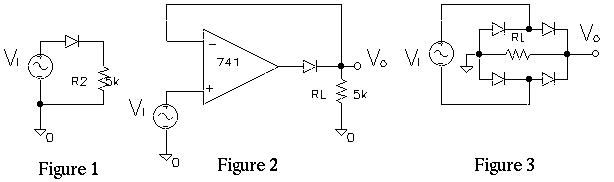EE 321 Lab

Lab 5: DIODES AND RECTIFIER CIRCUITS

Prelab for Lab 5

The purpose of this laboratory is to investigate the use of diodes in rectifier circuits and to build precision rectifiers.

Half Wave Rectifiers

1. Construct the simple half-wave rectifier shown in Figure 1 (use a 1N4001). Test its operation at 100 Hz for a range of input amplitudes, from to 20 V p-p down to 1 V p-p by superimposing the input and output waveforms on your scope. Describe results, sketch a sample waveform.2. How well does the rectifier work at higher frequencies (keep the input at 1 V p-p)? The undershoot that you observe at 100 kHz is caused by diode capacitance. Temporarily replace the rectifier diode (large capacitance) with a 1N4148 switching diode (low C). This should reduce the switching transient.

3. Replace the diode in your circuit with the `superdiode' shown in Figure 2 (use the fast diode). Repeat the above measurements, using a low input frequency (about 100 Hz).

Does this give better rectification, and why? Increase the signal frequency to about 2 kHz. What causes the delayed turn-on of the rectifier? (Hint: Look at the output of the op amp on the other trace.)

4. Substitute a 411 op amp. Compare the behavior of the two rectifiers at 2 kHz frequency. Why is the performance improved?

Full Wave Rectifiers

5. Construct the "bridge" rectifier (Figure 3) using a transformer to float the signal generator (10-20 V p-p, 100 Hz) and the 1N4001 diodes. Be sure to connect diodes as shown. Use a load of 5 k ohms.

6. Verify that the output is full-wave rectified. Copy the output wave form for 3 V p-p input. What is the flat part of the output waveform caused by? (Hint: How much voltage vin is required to turn the diodes on? Another hint: since the grounds of the signal generator and the output are different, both the input and output can NOT be viewed at once. The voltage between the positive side of the signal generator and rectifier ground is dificult to interpert.)

7. Place a 10 uF capacitor across the load resistance, being careful to observe the polarity of the electrolytic capacitor. The input signal should be 10 V p-p. Sketch output waveform and compare to the waveform without the filter capacitor. Measure the average DC voltage and peak voltage. Explain the waveform. Why is this called `filtering' the output?

8. Measure the p-p "ripple" on the output, both amplitude and frequency. How does the frequency compare to the input frequency? Compare the p-p ripple amplitude with its theoretical value.

9. Extra credit. Place the above rectifier circuit in the feedback loop of a non-inverting amplifier (as in Figure 12.38 in Sedra and Smith) to make a precision a.c. volt meter. Use a digital multimeter to measure the rectified filtered voltage instead of a current meter. The meter in 13.38 is replaced with a 5 k in parallel with a 10 uf capacitor, where the output is measured. Design the circuit so that 1 V rms input produces 1 V d.c. Sketch circuit diagram and test sensitivity.